# MapRuler Class

Draws a ruler on the map.

Namespace: DevExpress.Xpf.Map

Assembly: DevExpress.Xpf.Map.v22.2.dll

NuGet Package: DevExpress.Wpf.Map

## Declaration

``````public class MapRuler :
IMapRuler``````

The following members return MapRuler objects:

## Remarks

The Map Control uses rulers to measure distances and areas on a map. Two types of rulers are supported:

Distance Ruler Area Ruler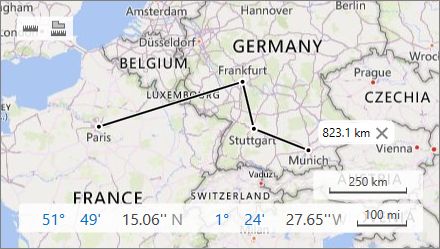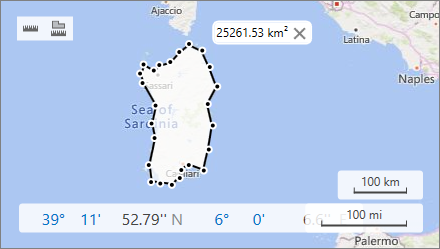### Create Rulers

Use the CreateRuler(RulerType, IList<CoordPoint>) method to add a ruler to the map. The following code displays a distance ruler:

``````MapRuler ruler = mapControl1.Measurements.CreateRuler(RulerType.Distance,
new List<CoordPoint>(){
new GeoPoint(48.864716, 2.349014),   // Paris
new GeoPoint(45.46427, 9.18951),     // Milan
new GeoPoint(48.20849, 16.37208) }); // Vienna
``````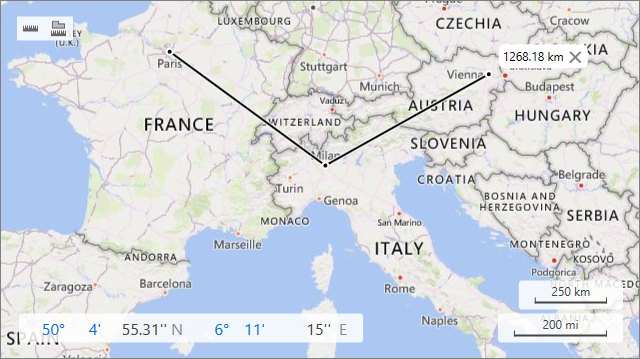### Configure Ruler Points

To modify a ruler, configure ruler points. You can use the following methods:

Name

Description

InsertPoint(MapRuler, CoordPoint, Int32)

Adds a new point to the ruler.

RemovePoint(MapRuler, Int32)

Removes the point with the specified index.

UpdatePoint(MapRuler, CoordPoint, Int32)

Changes the coordinates of the point with the specified index.

The example below creates a ruler to measure the distance between Paris, Milan and Vienna. After calculating this distance, the code updates the ruler as follows:

• a point is added at Berlin’s coordinates;
• the first point is moved from Paris to Dusseldorf;
• the point at Milan’s coordinates is removed from the ruler.
``````MapRuler ruler = mapControl1.Measurements.CreateRuler(RulerType.Distance,
new List<CoordPoint>() {
new GeoPoint(48.864716, 2.349014), // Paris
new GeoPoint(45.46427, 9.18951),   // Milan
new GeoPoint(48.20849, 16.37208)   // Vienna
});
//The code below updates the ruler.
mapControl1.Measurements.InsertPoint(ruler, new GeoPoint(52.520008, 13.404954), 2); // Berlin
mapControl1.Measurements.UpdatePoint(ruler, new GeoPoint(51.2217200, 6.7761600), 0); // Dusseldorf
mapControl1.Measurements.RemovePoint(ruler, 1); // Milan
``````
Before Update After Update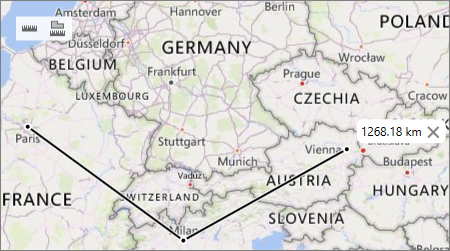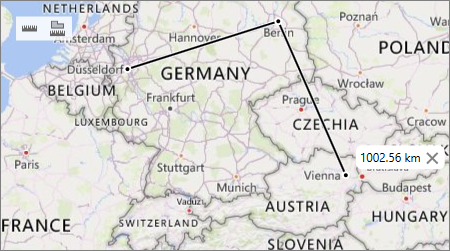### Remove Rulers

The RemoveRuler(MapRuler) method removes the ruler passed as a parameter.

``````mapControl1.Measurements.RemoveRuler(ruler);
``````

To delete all rulers from the map, call the RemoveRulers() method.

``````mapControl1.Measurements.RemoveRulers();
``````

Object
MapRuler